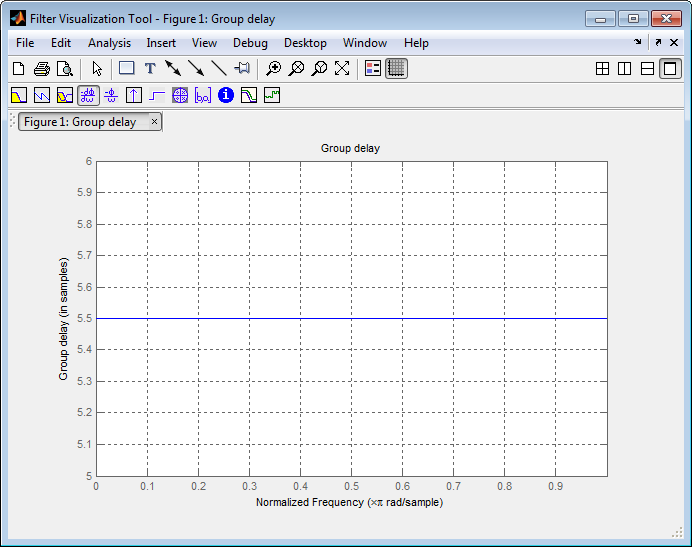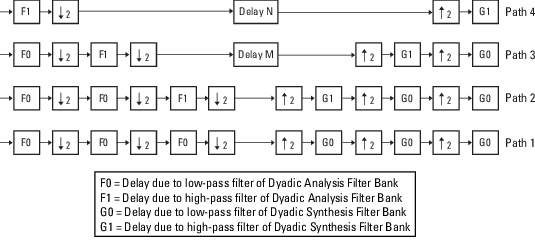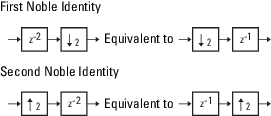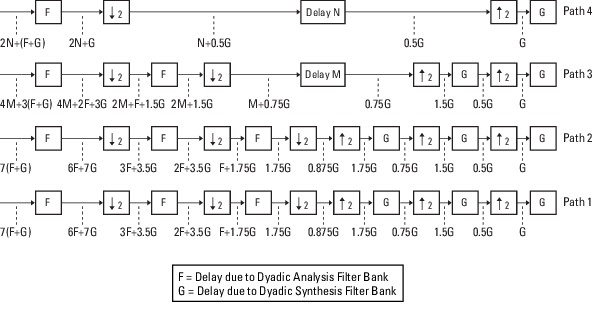## Calculate Channel Latencies Required for Wavelet Reconstruction

The following sections guide you through the process of calculating the channel latencies required for perfect wavelet reconstruction. This example uses the `ex_wavelets` model, but you can apply the process to perform perfect wavelet reconstruction in any model. To open the example model, type `ex_wavelets` at the MATLAB® command line.

Note

You must have a Wavelet Toolbox™ product license to run the `ex_wavelets` model.Before you can begin calculating the latencies required for perfect wavelet reconstruction, you must know the types of filters being used in your model.

The Dyadic Analysis Filter Bank and the Dyadic Synthesis Filter Bank blocks in the `ex_wavelets` model have the following settings:

• Filter = `Biorthogonal`

• Filter order [synthesis/analysis] = `[3/5]`

• Number of levels = `3`

• Tree structure = `Asymmetric`

• Input = ```Multiple ports```

Based on these settings, the Dyadic Analysis Filter Bank and the Dyadic Synthesis Filter Bank blocks construct biorthogonal filters using the Wavelet Toolbox `wfilters` function.

### Calculate the Group Delay of Your Filters

Once you know the types of filters being used by the Dyadic Analysis and Dyadic Synthesis Filter Bank blocks, you need to calculate the group delay of those filters. To do so, you can use the Signal Processing Toolbox™ fvtool.

Before you can use `fvtool`, you must first reconstruct the filters in the MATLAB workspace. To do so, type the following code at the MATLAB command line:

`[Lo_D, Hi_D, Lo_R, Hi_R] = wfilters('bior3.5')`

Where `Lo_D` and `Hi_D` represent the low- and high-pass filters used by the Dyadic Analysis Filter Bank block, and `Lo_R` and `Hi_R` represent the low- and high-pass filters used by the Dyadic Synthesis Filter Bank block.

After you construct the filters in the MATLAB workspace, you can use `fvtool` to determine the group delay of the filters. To analyze the low-pass biorthogonal filter used by the Dyadic Analysis Filter Bank block, you must do the following:

• Type `fvtool(Lo_D)` at the MATLAB command line to launch the Filter Visualization Tool.

• When the Filter Visualization Tool opens, click the Group delay response button () on the toolbar, or select Group Delay Response from the Analysis menu.

Based on the Filter Visualization Tool's analysis, you can see that the group delay of the Dyadic Analysis Filter Bank block's low-pass biorthogonal filter is 5.5.Note

Repeat this procedure to analyze the group delay of each of the filters in your model. This section does not show the results for each filter in the `ex_wavelets` model because all wavelet filters in this particular example have the same group delay.

### Reconstruct the Filter Bank System

To determine the delay introduced by the analysis and synthesis filter bank system, you must reconstruct the tree structures of the Dyadic Analysis Filter Bank and the Dyadic Synthesis Filter Bank blocks. To learn more about constructing tree structures for the Dyadic Analysis Filter Bank and Dyadic Synthesis Filter Bank blocks, see the following sections of the DSP System Toolbox™ User's Guide:

Because the filter blocks in the `ex_wavelets` model use biorthogonal filters with three levels and an asymmetric tree structure, the filter bank system appears as shown in the following figure.The extra delay values of M and N on paths 3 and 4 in the previous figure ensure that the total delay on each of the four filter paths is identical.

### Equalize the Delay on Each Filter Path

Now that you have reconstructed the filter bank system, you can calculate the delay on each filter path. To do so, use the following Noble identities:You can apply the Noble identities by summing the delay on each signal path from right to left. The first Noble identity indicates that moving a delay of 1 before a downsample of 2 is equivalent to multiplying that delay value by 2. Similarly, the second Noble identity indicates that moving a delay of 2 before an upsample of 2 is equivalent to dividing that delay value by 2.

The `fvtool` analysis in step 1 found that both the low- and high-pass filters of the analysis filter bank have the same group delay (F0 = F1 = 5.5). Thus, you can use F to represent the group delay of the analysis filter bank. Similarly, the group delay of the low- and high-pass filters of the synthesis filter bank is the same (G0=G1=5.5), so you can use G to represent the group delay of the synthesis filter bank.

The following figure shows the filter bank system with the intermediate delay sums displayed below each path.You can see from the previous figure that the signal delays on paths 1 and 2 are identical: 7(F+G). Because each path of the filter bank system has identical delay, you can equate the delay equations for paths 3 and 4 with the delay equation for paths 1 and 2. After constructing these equations, you can solve for M and N, respectively:

The `fvtool` analysis in step 1 found the group delay of each biorthogonal wavelet filter in this model to be 5.5 samples. Therefore, F = 5.5 and G = 5.5. By inserting these values into the two previous equations, you get M = 11 and N = 33. Because the total delay on each filter path must be the same, you can find the overall delay of the filter bank system by inserting F = 5.5 and G = 5.5 into the delay equation for any of the four filter paths. Inserting the values of F and G into 7(F+G) yields an overall delay of 77 samples for the filter bank system of the `ex_wavelets` model.

### Update and Run the Model

Now that you know the latencies required for perfect wavelet reconstruction, you can incorporate those delay values into the model. The `ex_wavelets` model has already been updated with the correct delay values (M = 11, N = 33, Overall = 77), so it is ready to run.After you run the model, examine the reconstruction error in the Difference scope. To further examine any particular areas of interest, use the zoom tools available on the toolbar of the scope window or from the View menu.### References

 Strang, G. and Nguyen, T. Wavelets and Filter Banks. Wellesley, MA: Wellesley-Cambridge Press, 1996.

## SupportGet trial now